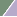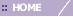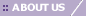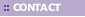# Manhattan

Overview

The Manhattan distance function computes the distance that would be traveled to get from one data point to the other if a grid-like path is followed. The Manhattan distance between two items is the sum of the differences of their corresponding components.

The formula for this distance between a point X=(X1, X2, etc.) and a point Y=(Y1, Y2, etc.) is: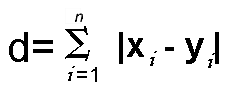Where n is the number of variables, and Xi and Yi are the values of the ith variable, at points X and Y respectively.

The following figure illustrates the difference between Manhattan distance and Euclidean distance: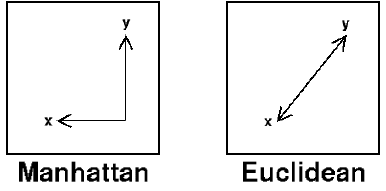Related Topics:

Distance Metrics Overview